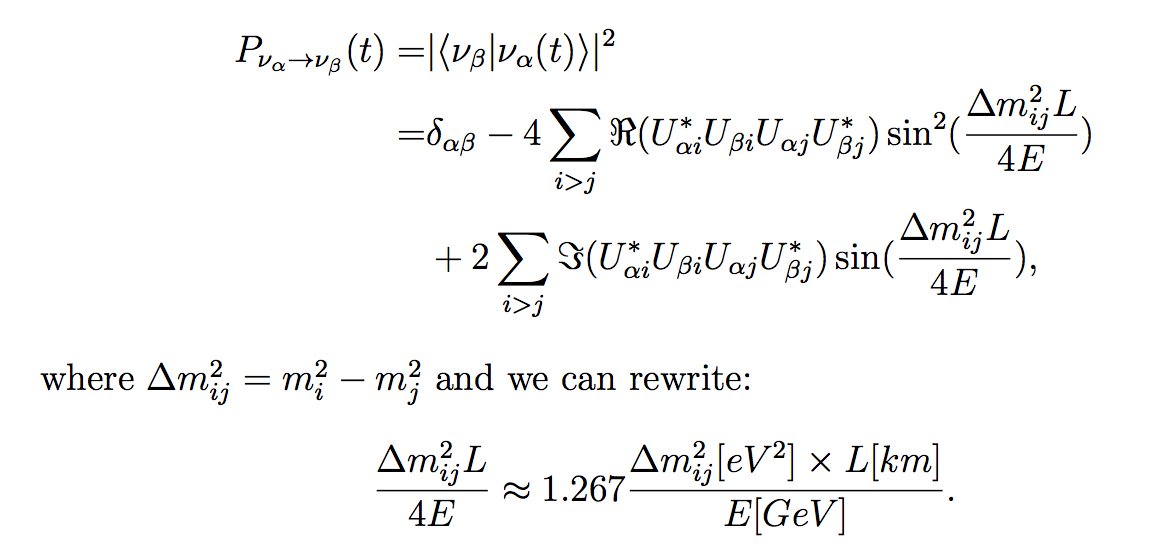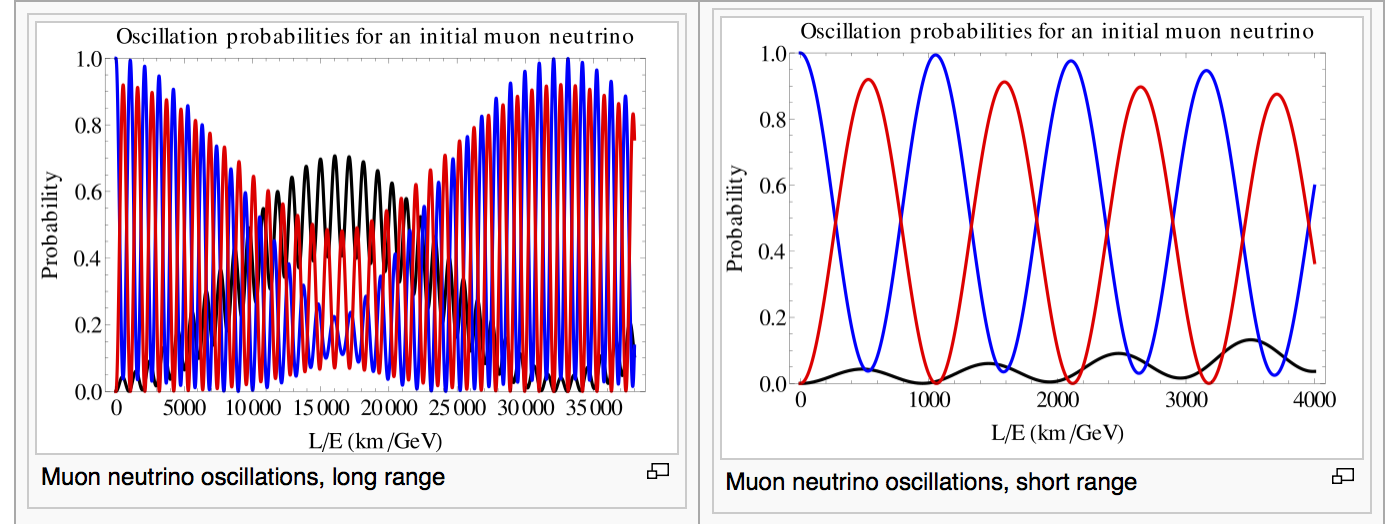English | Tiếng Việt

Introduction to Neutrino Oscillations

Neutrino oscillation, now a well-understood phenomenon, indicates that neutrinos have mass, and their flavor eigenstates are different from their mass eigenstates. Neutrino oscillations provide the first evidence of physics be- yond the Standard Model of the elementary particles. Over eight decades, our picture of neutrino physics has been revolutionized, but some fundamental questions still remain unanswered.

Neutrino oscillations spring from a quantum mechanical mixing between the mass and flavor eigenstates of neutrinos. Neutrinos, which are observed in the experiments, are created with other fermions through weak nuclear force, which does not change the flavor of the particle. The νe, νμ and ντ are labeled as the flavor eigenstates of neutrinos (generalized as να). The only way flavor eigenstates of neutrinos can be constructed to be invariant under weak nuclear force is a mixture of exactly the right portion of the three flavor-mixed eigenstates ν1, ν2 and ν3. The flavor eigenstates can be derived from the mass eigenstates with a leptonic mixing matrix Uαi, so-called PMNS mixing matrix. The probability of observing a neutrino of flavor β (νβ) at time t (equivalent to distance L) from a neutrino of original flavor α (να) is then given by:Following graph is examples of oscillation probability from an initial muon (flavor) neutrino to other flavors (electron,  muon, tau) neutrinos.Electron neutrino oscillations, long range. Here and in the following diagrams black means electron neutrino, blue means muon neutrino and red means tau neutrino. Credit: Wikipedia

How can we measure neutrino oscillations?
To measure neutrino oscillations, at first we need a source of neutrino in which neutrino flavor components (number of electron neutrinos, muon neutrino, tau neutrinos) are well-understood. It can be from nature (the sun, atmospheric, supernova, etc..) or man-made source (accelerator, accelerator, etc..). In principle, one large detector, which is placed at the location such that the considering oscillation probability is almost maximum, is enough. However, an additional detector placed near the neutrino source (so-called near detector, and the previous one is so-called far detector) is often necessary to understand the neutrino flux before neutrino oscillates.This is so-called two-detector baseline paradigm. In current long-baseline neutrino experiments, T2K and NOvA uses far detector at 295km and 810km away from the neutrino source respectively. These optimal baselines depends mainly on energy of neutrino flux and target of the neutrino physics.

There are two conventional ways to measure neutrino oscillation: disappearance and appearance.
• Disappearance: to see how much neutrino of original flavor α (να) reduces after traveling a defined distance. For example, you have a beam of 𝜈𝜇  and you predict that you will see N𝜇 neutrino events of classified 𝜈𝜇 in detector. However what you observed is M𝜇 events, which is smaller the predicted N𝜇. In that case, we say, 𝜈𝜇 is deficit or 𝜈𝜇 is disappeared.
• Appearance: to see a how much neutrino of new flavor β (νβ) is appeared from a defined neutrino να flux (𝛽≠α). For example, you have a beam of 𝜈𝜇  and you predict that you will see Ne event of 𝜈e in your detector (can be from intrinsic beam- there is no beam with 100% 𝜈𝜇, or from misclassified event ). However you observe Me events of classified 𝜈e event, which is larger the predicted Ne. In this case, we say 𝜈 is appeared.

It is reminded that the neutrino oscillation probability depends on its energy. Thus for precise measurement, it is necessary to reconstruct energy for each neutrino event and present disappearance or appearance as a function of energy. These pattern then is fitted with the oscillation probability formulas to extract the neutrino oscillation parameters.

Present neutrino oscillation landscape:Snapshot from S. Cao’s slide at PASCOS 2016

Go to >>> Introduction to Neutrino Physics
Go to >>>Neutrino Detection
Go to >>>CP violation search
Go to >>>Sterile neutrino search

Back >>>>HOME
--------------------------------
"Long-baseline News is good channel to get updated information in neutrino physics.
http://www.hep.anl.gov/ndk/longbnews/
To receive the (~100 line) newsletter each month, the listname is "long-bnews". You can subscribe by sending a message "subscribe long-bnews firstname lastname" to listserv@fnal.gov . Put your name, not your email, and put it in the body of the message, not the subject.

*This site is kindly hosted by HEP Lab, Kyoto University